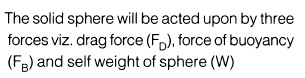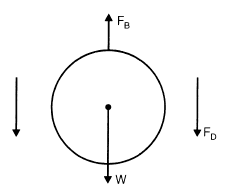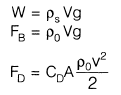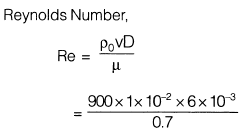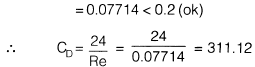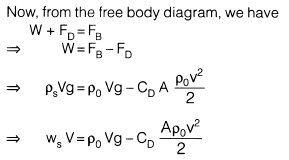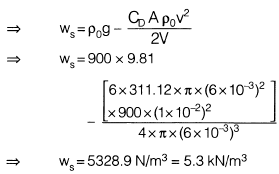Courses

# Test: Fluid Mechanics- 2

## 20 Questions MCQ Test GATE Civil Engineering (CE) 2022 Mock Test Series | Test: Fluid Mechanics- 2

Description
This mock test of Test: Fluid Mechanics- 2 for GATE helps you for every GATE entrance exam. This contains 20 Multiple Choice Questions for GATE Test: Fluid Mechanics- 2 (mcq) to study with solutions a complete question bank. The solved questions answers in this Test: Fluid Mechanics- 2 quiz give you a good mix of easy questions and tough questions. GATE students definitely take this Test: Fluid Mechanics- 2 exercise for a better result in the exam. You can find other Test: Fluid Mechanics- 2 extra questions, long questions & short questions for GATE on EduRev as well by searching above.
QUESTION: 1

### What is the absolute pressure at A measured by an open tube manometer as shown in the figure below? (Assume atmospheric pressure as 103 kN/m2; SA and SB are the specific gravities of the two fluids)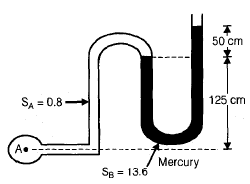Solution: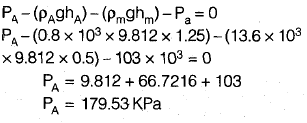QUESTION: 2

### The coefficient of contraction for an orificemeter is 0.74. Area of throat of orificement is 95.6 mm2. Find the value of diameter of vena-contracta formed in this pipe and orificemeter arrangement

Solution: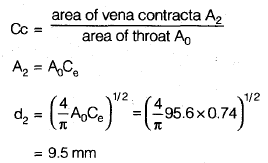QUESTION: 3

### For the given pipe length with area variation the total energy line and hydraulic line will be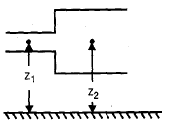Solution:
QUESTION: 4

The values of friction factor in mis judge by +25% in using Darcy equation for the same head loss the error in discharge calculation will be

Solution: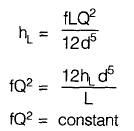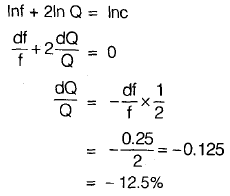QUESTION: 5

The separation of boundary layer is a phenomenon in which the boundary layer gets detached from the wall due to progressive deceleration of flow. The boundary layer separation occur when

Solution: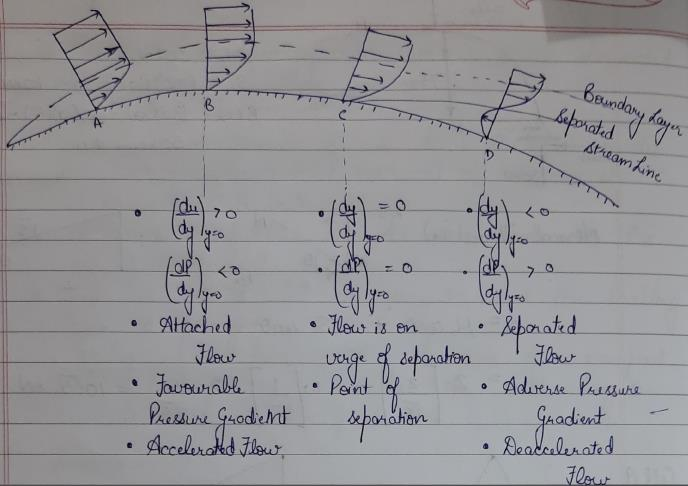QUESTION: 6

The velocity distribution in laminar flow through pipe follows a parabolic law altering a maximum velocity at the pipe axis. The Hagenpoiseuille equation which given this type of flow may be expressed as

Solution:
QUESTION: 7

A pipe line carrying water has surface protrusions of average height 0.10 mm. If the shear stress developed is 7.85 N/m2, then the pipe surface will act as

Solution: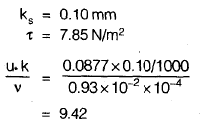Since roughness value lies between 4 and 100 the pipe act as in transition.

QUESTION: 8

A water jet of 5 cm diameter moves with a constant velocity of 20 m/s and strikes a stationary and symmetrical curved plate at the centre. If the jet is deflected through an angle of 120° at the inlet and of the current plate, the force exceeded by the water jet in the direction of jet will be

Solution: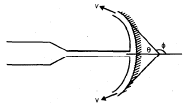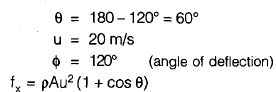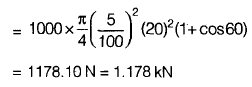QUESTION: 9

A centrifugal pump is required to pump water to an open water tank situated 4 km away from the location of the jump through a pipe of diameter 0.2 m having Darcy’s friction factor of 0.01. The average speed of water in the pipe is 2 m/s. If it is to maintain a constant head of 5 m in the tank, neglecting other minor losses, the absolute discharge pressure at the pump exit is

Solution: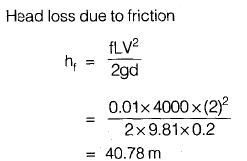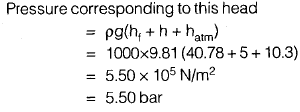QUESTION: 10

A Francis turbine working at 400 rpm has a unit speed of 50 rpm and develops 500 kW of power. What is the effective head under which this turbine operates?

Solution: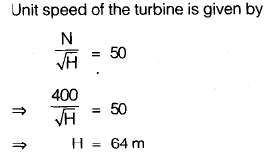QUESTION: 11

Water flows through a horizontal conical pipe 12 m long and having a diameter of 20 cm at the inlet and 15 cm at the discharge end. A constant discharge of 40 lit/sec through the pipe. The loss of head due to pipe friction will be (f = 0.04)

Solution: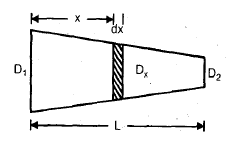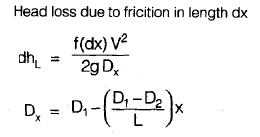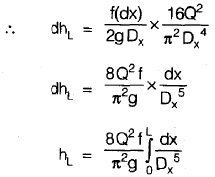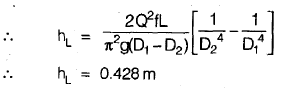QUESTION: 12

A single stage centrifugal pump has an impeller of 30 cm diameter which rotates at 1780 rpm and discharge 4000 lit/min to a height of 25 m at an efficiency of 70% the no. of stages required of a multistage similar pump to lift 5000 lit/min to 175 m at 1500 rpm will be

Solution: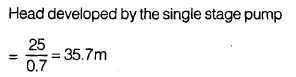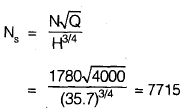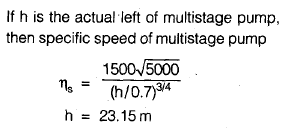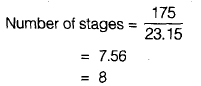QUESTION: 13

A Kaplan turbine produces 38,700 HP while working under a head of 11.75 m. The turbine is set such that the inlet of the draft tube is 0.65 m below the tail race level. The draft tube inlet and outlet areas are 38m2 and 54.7 m2. Assuming a turbine efficiency of 0.90 and draft tube efficiency of 0.79, the pressure at the inlet to the draft tube, in meters of water is

Solution:
QUESTION: 14

A centrifugal pump is discharging 4500 lit/sec and operates at 1800 rpm against a head of 35 m. The power input to the pump is 3 kW. If this pump is required to operate at 1200 rpm, what will be the power input to the pump?

Solution: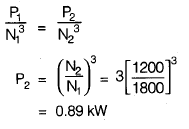QUESTION: 15

A conical draft tube having diameter at the top as 2 m and pressure head at 7 m of water (vacuum), discharges water at the outlet with a velocity of 1.2 m/s at the rate of 25 m3/s. If atmospheric pressure head is 10.3 m of water and losses between the inlet and outlet of the draft tubes are negligible, find the length of draft tube immersed in water. Total length of tube is 5m,

Solution: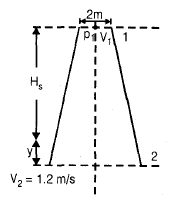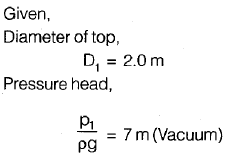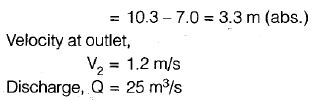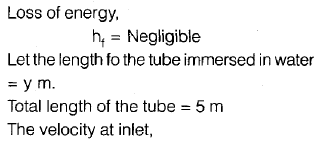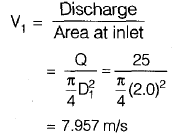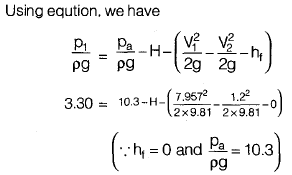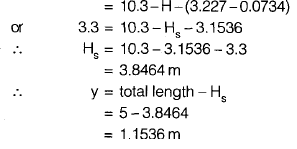QUESTION: 16

Find the power required to tow length wise a plate 1.2 m wide and 3 m long at a velocity of 2.4 m/s in water at 23°C (νwater = 0.9 × 10−6 m3/s)

Solution: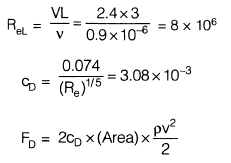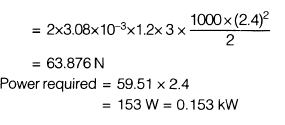QUESTION: 17

2 pipes of equal length are connected in series. The diameter of second pipe is two times that of first pipe. Ratio of frictional head loss of first to that of second will be

Solution: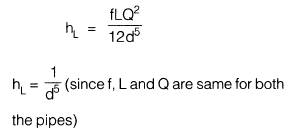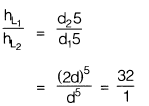QUESTION: 18

A pressure gauge is installed in front of a valve on a water pipe of diameter D = 50 mm. When the valve is closed the gauge reads 6 atm. When the valve is opened the reading drops to 2 atm. The rate of discharge of water in the pipe line will be

Solution: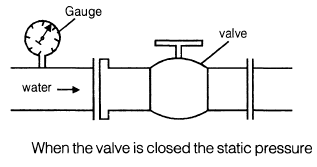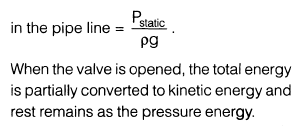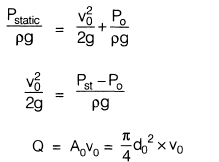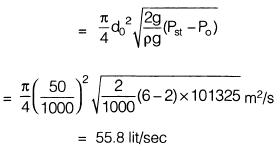QUESTION: 19

A sliding gate 3 m wide and 1.5 m high lies in vertical plane and has a coefficient of friction of 0.20 between itself and guides. If the gate weights 19.6 kN and if upper edge is at a depth of 10 m then the vertical force required to raise the gate will be

Solution: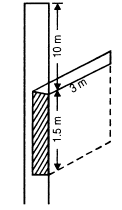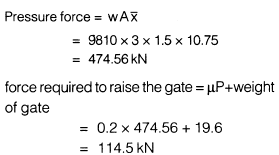QUESTION: 20

A solid sphere (diameter 6 mm) is rising through oil (mass density 900 kg/m3, dynamic viscosity 0.7 kg/m-s) at a constant velocity of 1 cm/s. What is the specific weight of the material from which the sphere is made? (Take g = 9.81 m/s2)

Solution: